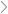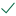Questions & AnswersStatistics and ProbabilityUse a significance level of .05 for all analyses.   A researcher...

QuestionAnswered step-by-step

# Use a significance level of .05 for all analyses.   A researcher...

Use a significance level of .05 for all analyses.

A researcher wanted to determine whether showing a word upside down versus right side up affects memory of the word.  Thirty students were randomly divided into two groups.  One group were shown a list of words right side up, the other group were shown the same list of words upside down.  The number of words each student could recall from the list was recorded.  What type of t test should be used the analyze the data?

A. t test for independent groups

B. t test for dependent groups.

A researcher wanted to determine whether meditation affects heart rate.  The heart rates of thirty students were measured, the students then meditated for 30 minutes, and their heart rates were measured again (two scores per person).  What type of t test should be used the analyze the data?

A. t dependent groups

B. t independent groups

A researcher wanted to determine whether meditation affects heart rate.  The heart rates of thirty students were measured, the students then meditated for 30 minutes, and their heart rates were measured again (two scores per person).  What type of design is this?

A.repeated measures

B.true experimental

C.quasi-experimental

D.matched-pairs

A researcher wanted to determine whether meditation affects heart rate.  The heart rates of thirty students were measured, the students then meditated for 30 minutes, and their heart rates were measured again (two scores per person).  What is the independent variable? What is the dependent variable?

A. before vs. after meditation

B. heart rate

A researcher wanted to determine whether meditation affects heart rate.  The heart rates of thirty students were measured, the students then meditated for 30 minutes, and their heart rates were measured again (two scores per person).  What would be the appropriate H0 for this experiment?

A.H0: μBefore = μAfter

B.H0: μBefore ≠ μAfter

C.H0: μD=0

D.H0: μD≠0

A researcher wanted to determine whether meditation affects heart rate.  The heart rates of thirty students were measured, the students then meditated for 30 minutes, and their heart rates were measured again (two scores per person).  What would be the appropriate Ha for this experiment?

A.Ha: μD≠0

B.Ha: μBefore ≠ μAfter

C.Ha: μD=0

D.Ha: μBefore = μAfter

If the null hypothesis is true, then the average value for MD would equal?

A researcher wanted to determine whether meditation affects heart rate.  The heart rates of four students were measured, the students then meditated for 30 minutes, and their heart rates were measured again (two scores per person).  The data are given below:

MD = ?

A researcher wanted to determine whether meditation affects heart rate.  The heart rates of four students were measured, the students then meditated for 30 minutes, and their heart rates were measured again (two scores per person).  The data are given below:

SSD = ?

A researcher wanted to determine whether meditation affects heart rate.  The heart rates of four students were measure, the students then meditated for 30 minutes, and their heart rates were measured again (two scores per person).  The data are given below.  To keep you from having to compute everything from the beginning again the SSDwill be given.

SSD=18.75

est. σMD = ?

A researcher wanted to determine whether meditation affects heart rate.  The heart rates of four students were measure, the students then meditated for 30 minutes, and their heart rates were measured again (two scores per person).  The data are given below. To keep you from having to compute everything from the beginning the values of MD and est. σMD will be given.

MD=2.25

est. σMD = 1.25

t = ?

A researcher wanted to determine whether meditation affects heart rate.  The heart rates of five students were measure, the students then meditated for 30 minutes, and their heart rates were measured again (two scores per person).  The data are given below:

df = ?

In which of the following analyses would you reject H0?  (select all that apply)

A.t(3)=-0.93,p=.4

B.t(12)=2.35, p=.037

C.t(12)=0.14, p=.89

D.t(35)=-2.87, p=.007

Assuming you have no serious confounding variables.  In which of the following analyses would you conclude that the independent variable had an effect on the dependent variable? (select all that apply)

A.t(15)=-1.94, p=.071

B.t(6)=2.63, p=.039

C.t(4)=1.62, p=.18

Assuming you have no serious confounding variables.  In which of the following analyses would you conclude that the independent variable had an effect on the dependent variable? (select all that apply)

A.t(24)=-2.10, p=.046

B.t(7)=1.31, p=.23

C.t(14)=-1.85, p=.086

A study consists of one independent variable with five levels (i.e. there were five groups in the experiment). A total of 50 subjects were randomly divided into the five groups (10 in each group).  What would be the appropriate H0 for this experiment?

A.H0: μ1 = μ2

B.H0: μ1 = μ2 = μ3 = μ4 = μ5

C.H0: μ1 = μ2 = μ3 = μ4

D.H0: M1 = M2 = M3 = M4 = M5

A study consists of one independent variable with five levels (i.e. there were five groups in the experiment). A total of 50 subjects were randomly divided into the five groups (10 in each group).  What would be the appropriate Ha for this experiment?

A.Ha: μ1 ≠ μ2 ≠ μ3 ≠ μ4 ≠ μ5

B.Ha: at least one μ is different than the rest

C.Ha: at least one M is different than the rest

If H0 is true, what do we expect the value of F to be?

A.F > 1.00

B.F < 1.00

C.F ≈ 1.00

A study consists of one independent variable with four levels (i.e. there were four groups in the experiment). A total of 40 subjects were randomly divided into the four groups (10 in each group).

Given the following summary table

F= ?

In some experiment, F(4,30)=2.93, p=.037

A.Reject H0

B.Do not reject H0

In some other experiment F(2,55)=1.61, p=.21

A.We can conclude that a statistically significant difference exists somewhere among the group means.

B.We cannot determine whether or not a statistically significant difference exists somewhere among the group means.

In yet another experiment; F(3,21)=1.03, p=.40

A.We can conclude that all of the population means differ.

B.We can conclude that at least one population mean is different than the rest.

C.We cannot determine whether or not at least one of the population means is different than the rest.

In some other experiment F(5,15)=4.56, p=.01

Assuming we have no serious confounding variables...

A.We cannot determine whether or not the independent variable had an effect somewhere among the groups.

B.We can conclude that the independent variable had an effect somewhere among the groups.

A study consists of one independent variable with five levels (i.e. there were five groups in the experiment). A total of 50 subjects were randomly divided into the five groups (10 in each group).

If the means of the five groups were:  M1=30, M2=27, M3=33, M4=23, M5=25, can we conclude that μ1 ≠ μ2?

A. no

B. yes

Which type of error is of most concern when running many comparisons in an ANOVA?

A.Not rejecting H0 when H0 is false.

B.Rejecting H0 when H0 is true.

In general, the various comparisons procedures keep the number of errors under control when you are performing many comparisons by...

A.Making it harder to reject H0 for each comparison.

B.Making it easier to reject H0 for each comparison.

Which of the following are valid comparisons in an experiment that has four groups  (G1,G2,G3, and G4)? Select ALL that apply

A.(G1 & G3) vs. (G2)

B.(G1 & G3) vs. (G2 & G4)

C.G1 vs G3

D.G1 vs. G2 vs. G3

E.G1 vs (G1 & G3)

A researcher plans on running 3 comparisons using Dunn's Method (Bonferroni t). What significance level would be used for each comparison?

A study consists of one independent variable with five levels (i.e. there were five groups in the experiment). A total of 50 subjects were randomly divided into the five groups (10 in each group).  The Fcriticalfor the overall F test is Fcritical = 2.58.  What would the FScheffe value for determining whether or not a comparison is significant using Scheffe's method?

FScheffe = ?

In a design with two independent variables how many F tests can be performed?

In a design with 2 independent variables, one variable having three levels, and the other variable having six levels, how many different treatment combinations ('cells') will there be?

In a study students were asked to rate the desirability of two different foods: fresh strawberries or raw steaks. Half the students were hungry and half the students were full when making the judgment. The researcher found that hungry students preferred strawberries while the full students preferred steaks. Which of the following describes this result.

Level of hunger and type of food interact.

Level of hunger and type of food do not interact.

Look at the following table of cell, row, and column means. As with the homework assignment and quiz, if there is any apparent effect at all say that there appears to be an effect.

Row, Column, and Cell Means

There is an apparent effect of B

There is an apparent interaction of A and B

There is an apparent effect of A

Look at the following table of cell, row, and column means. As with the homework assignment and quiz, if there is any apparent effect at all say that there appears to be an effect.

Row, Column, and Cell Means

There is an apparent effect of B

There is an apparent effect of A

There is an apparent interaction of A and BSolved by verified expert

se
sectetur adipiscing elit. Nam lacinia pulvinar tortor nec fasectetur adipiscing elit. Namsectetur adipiscing elit. Nam lacinia pulvinar t
sectetur adipiscing elit. Nam lacinia pulvinar tortor nec facilisis. Pellentesque dapibus efficitur laoreet. Nam risu

sectetur adipiscing elit. Nam lacinia pu10,000 step-by-step explanations

## Step-by-step explanation

sectetur